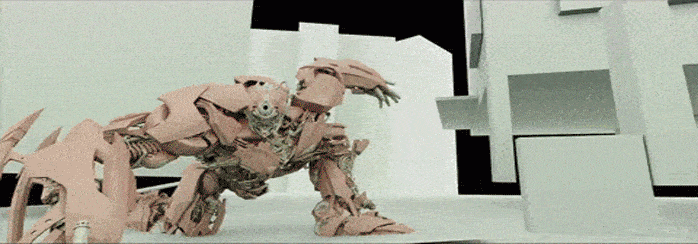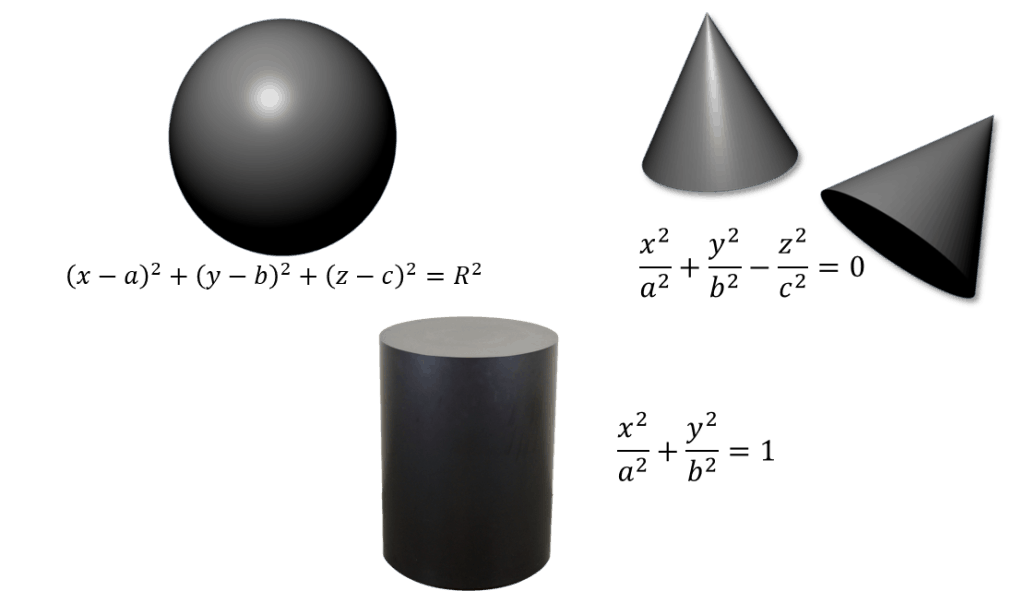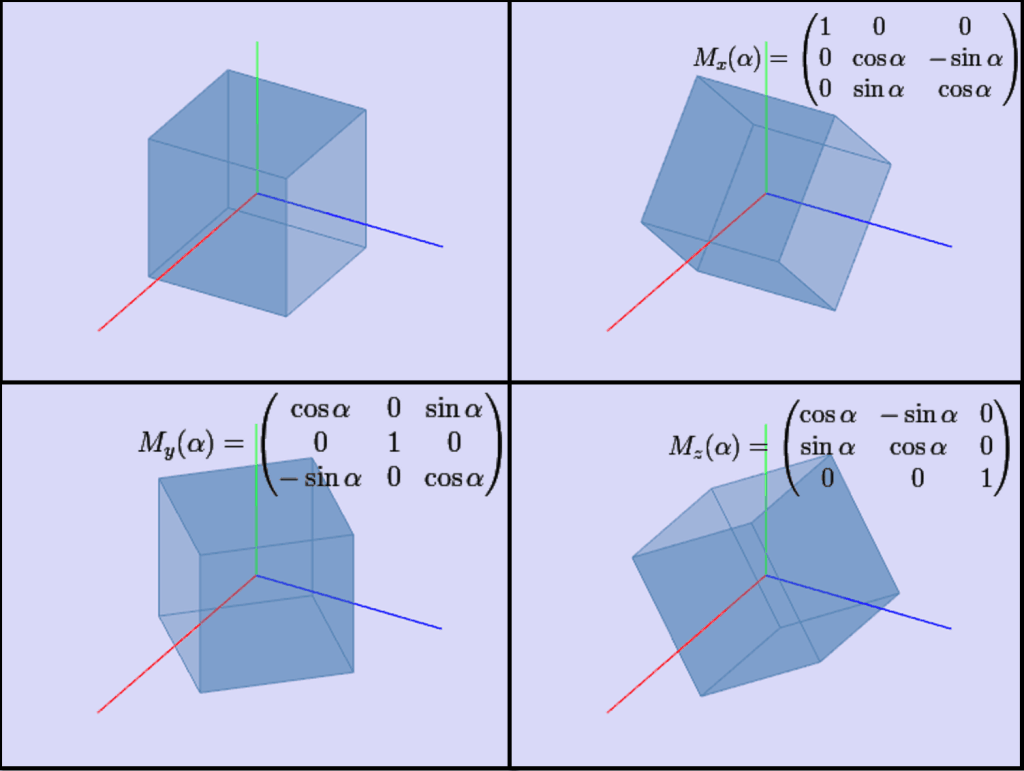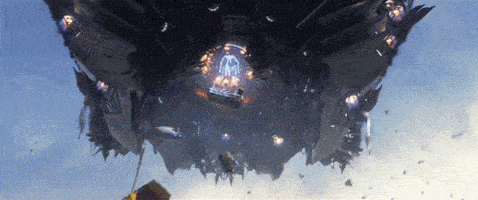# How Math Is Used in 3D Computer GraphicMost high school students are afraid of math lessons wondering whether they can ever use the acquired knowledge in the real life. Today, when so many calculations are carried out by computers, algebra and geometry find good realization in 3D graphics industry. And if you still don’t know how why math is so useful for 3D graphics, this article is for you.

In the 21st century, 3D graphics is frequently used in the movies, cartoons, games, etc. If previously, mathematical methods allowed to show simple models with primitive movements, now, mathematical advances help to create much more human-like characters with realistic facial expressions and to apply various visual special effects. Let’s now take a closer look at how the math works in 3D.

We’ll start with the fact that the working process in any 3D program (Maya, 3D Max, Cinema 4D, Blender) presupposes the use of the coordinate system, which is familiar to us from school classes. You perfectly know (or at least have heard about) the term of a vector, learn to find the length of the segment, as well as determine the coordinates of the point. Those seem boring tasks, but they can be transformed into beautiful things:

Each point of the object has its own coordinate $(x_{i},y_{i},z_{i})$; the distance between the two points is calculated with the formula $\sqrt{(x_{i}-x_{j})^2+(y_{i}-y_{j})^2+(x_{i}-x_{j})^2}$. If you want to increase or decrease the size of an object, you should recall a formula of multiplying a vector by a number $\lambda \cdot (x, y, z)=(\lambda \cdot x, \lambda \cdot y, \lambda \cdot z)$. Actually, nothing new, huh?

Let’s move on. Standard objects like cube, sphere, cylinder, and so on, which you often meet in geometry, are widely used for modeling in these programs.And here is a set of integral actions: turning and moving the object. In this case, the matrices, which you know from the linear algebra, start working. The addition and subtraction, multiplication, transposition operations with matrices often bring some difficulties to your homework or final tests. But the meaning and visual interpretation of these actions are very simple:Of course,  the objects and operations above are only a small part of mathematics used in 3D graphic programs. More complex activities in 3D need the knowledge of such fields as Calculus, Differential Geometry, Numerical Methods, Sampling Theory and Signal Processing, Probability and Statistics and, etc. So as you see, despite all these formulas are inscribed in the 3D packages program code, the knowledge and understanding of different math fields will be a good assistant in creating a three-dimensional masterpiece.Thus, if you have decided to connect your future profession with 3D graphics, you are on the right way. Therefore, we advise you to do all your math homework carefully and in case you have any questions, you can always ask us or watch our video tutorials.

94 Shares
Filed under Math.